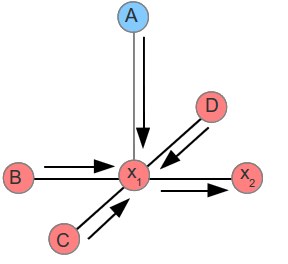# Loopy BP: an easy implementation on Pregel Model

Pregel: Message Passing.

Focus on the process, no matter each vertex computation.

Steps :

(this part was referenced from a blog)

A node passes a message to an adjacent node only when it has received all incoming messages, excluding the message from the destination node to itself. Below shows an example of a message being passed from  x1 to x2 .Node x1  waits for messages from nodes A,B,C,D before sending its message to . As a reminder, it does not send the message from  x2->x1 back to x1 (no feedback) .

An easy version of algo given by me:

Input: Graph g, number of iteration n.

Output: the “best” label for each vertex.

_________________________________________

function LoopyBP (Graph g, int n):

for each vertex in Graph g:

initialise message.

for each edge in Graph g:

initialise conditional probability.

// to avoid wait-forever problem ( a random state can be chosen, intend to testing)

for n times of iteration:

(simultaneously) pass message to the peer node(?)

calculate dot-product

find the best label as the belief

_________________________________________

Five key questions

1. message init

for each vertex, we can have multiple states with a random probability of each, but sum up to 1(normalisation).

etc. (0.3,0.6,0.1)

2. edge init

for each edge, init, same as question 1.

3. message update

when sending message, if A->B

message (A->B) =( Neighbours exclude B Sum-up in Vertex A )* Edge (A->B)

4. calculate belief

after received every message from peer vertex,

choose the best label for the node, marked as the belief

5. loops

do BP for many times.